# BP神经网络

## 神经元模型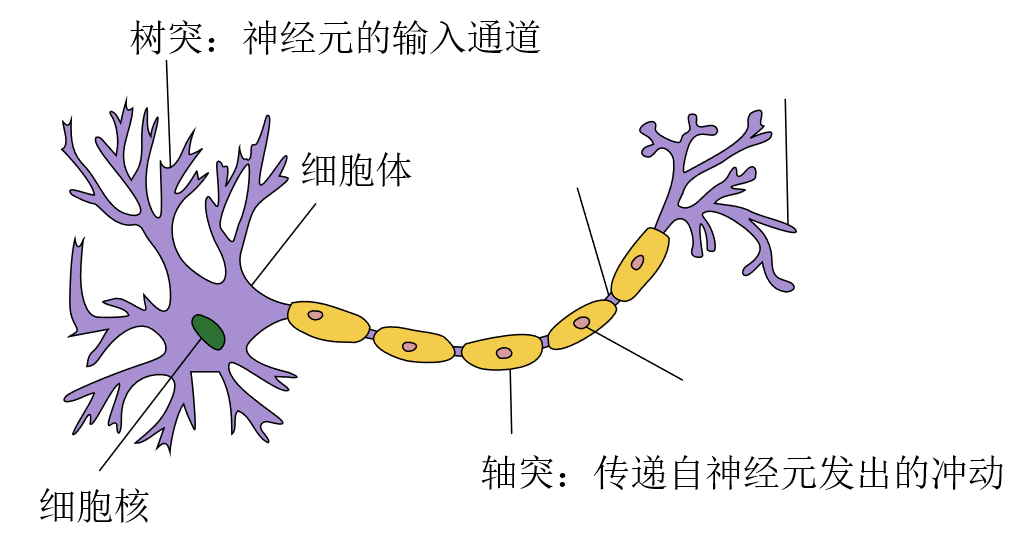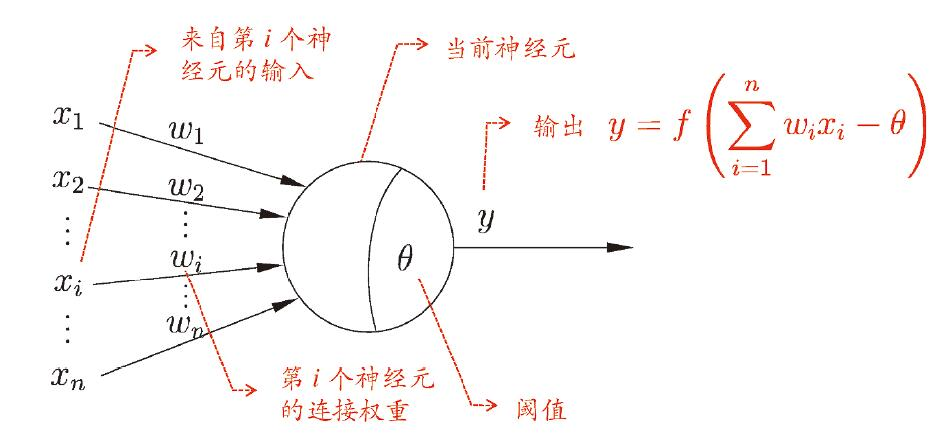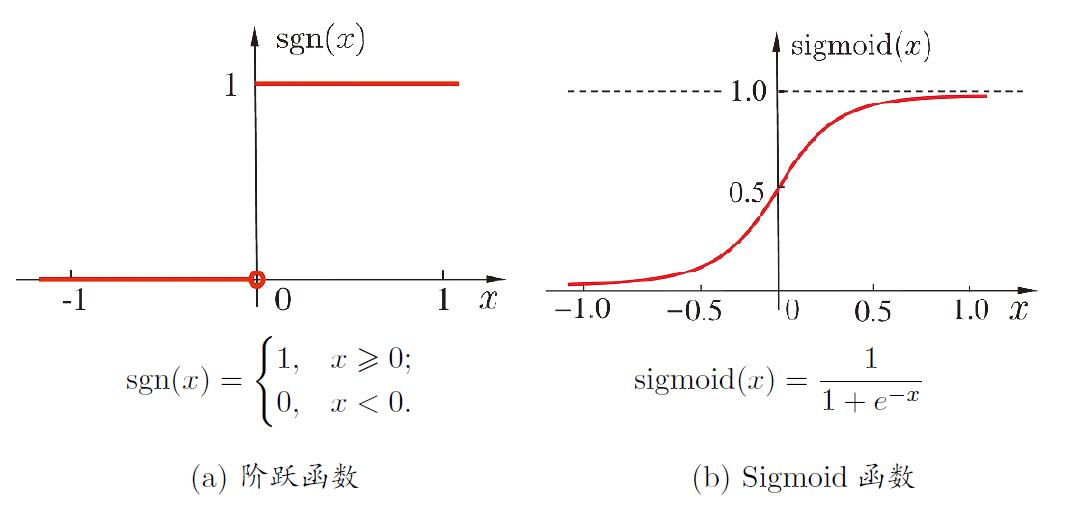$Sigmoid$函数也存在缺点，当输入的绝对值大于某个阈值时会过快进入饱和状态，使梯度趋于0，模型收敛缓慢。

$ReLU$函数在一些现代神经网络中也很常用，它是一个很简单的分段线性函数

## 感知机与多层网络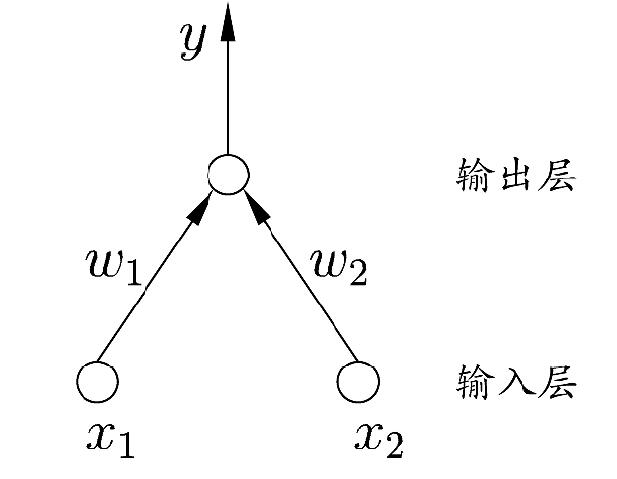$x_1$ $x_2$ $y=f(1⋅x_1+1⋅x_2-2)$ $y=f(1⋅x_1+1⋅x_2-0.5)$ $y=f(-0.6⋅x_1+0⋅x_2+0.5)$
0 0 0 0 1
0 1 0 1 1
1 0 0 1 0
1 1 1 1 0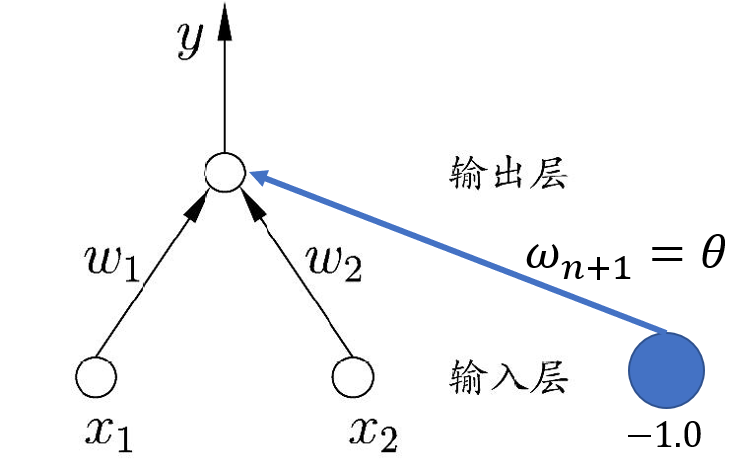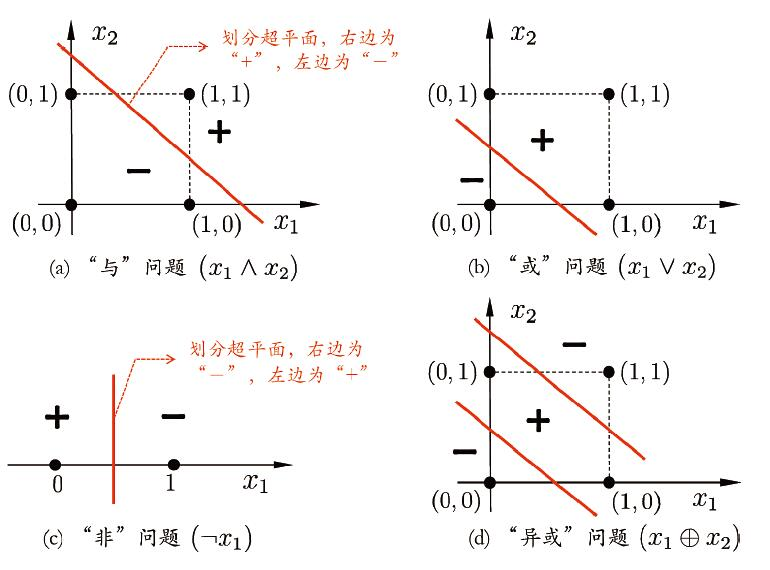$x_1$ $x_2$ $y^{(1)}=f(x_1+x_2-0.5)$ $y^{(2)}=f(-x_1-x_2+1.5)$ $y=y^{(1)}+y^{(2)}-1.5$
0 0 0 1 0
0 1 1 1 1
1 0 1 1 1
1 1 1 0 0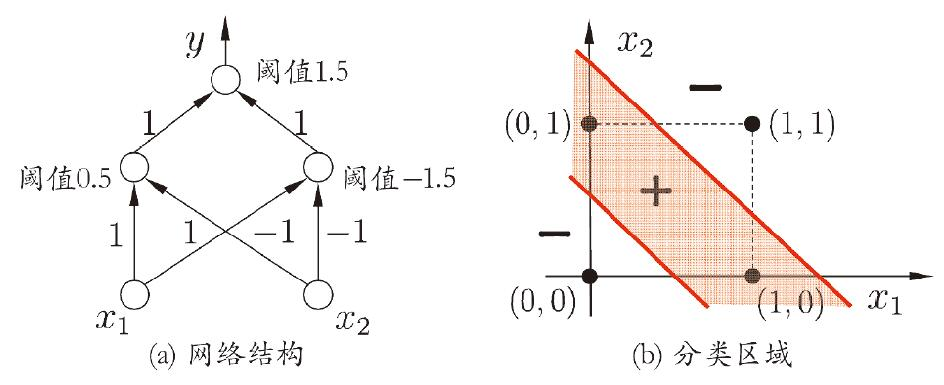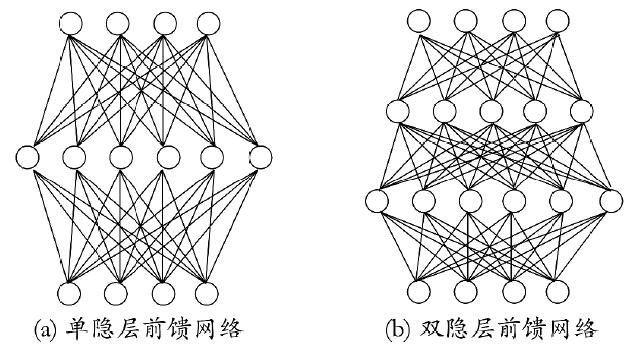## 误差反向传播算法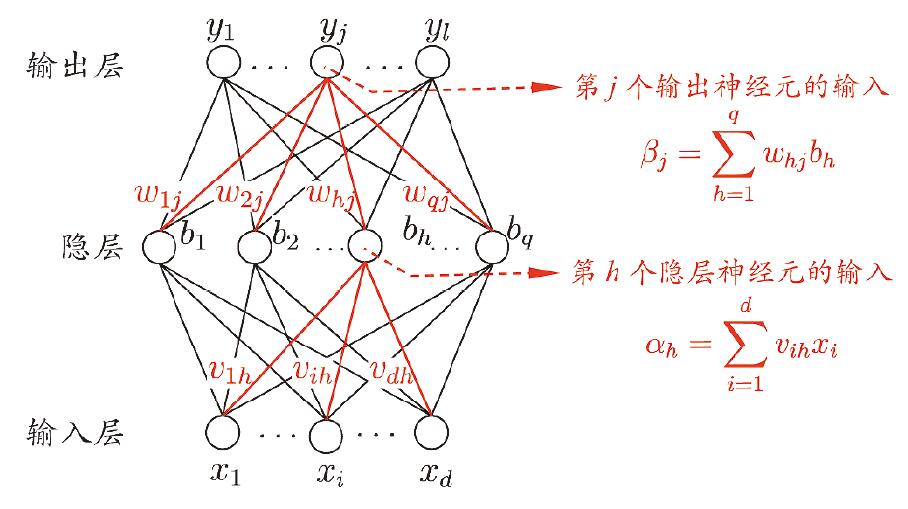$d$ 输入神经元个数
$l$ 输出神经元个数
$q$ 隐层神经元个数
$\theta_j$ 输出层第$j$个神经元的阈值
$γ_h$ 隐层第$j$个神经元的阈值
$v_{ih}$ 输出层第$i$个神经元与隐层第$h$个神经元间的连接权
$ω_{hj}$ 隐层第$h$个神经元与输出层第$j$神经元间的连接权
$\alpha_h$ 隐层第$h$个神经元接收到的输入
$\beta_j$ 输出层第$j$个神经元接收到的输入
$b_h$ 隐层第$h$个神经元的输出

• 输入层到隐层的$d×q$个权值

• 隐层到输出层的$q×l$个权值

• 隐层神经元的$q$个阈值

• 输出层神经元的$l$个阈值

$Sigmoid$的导函数：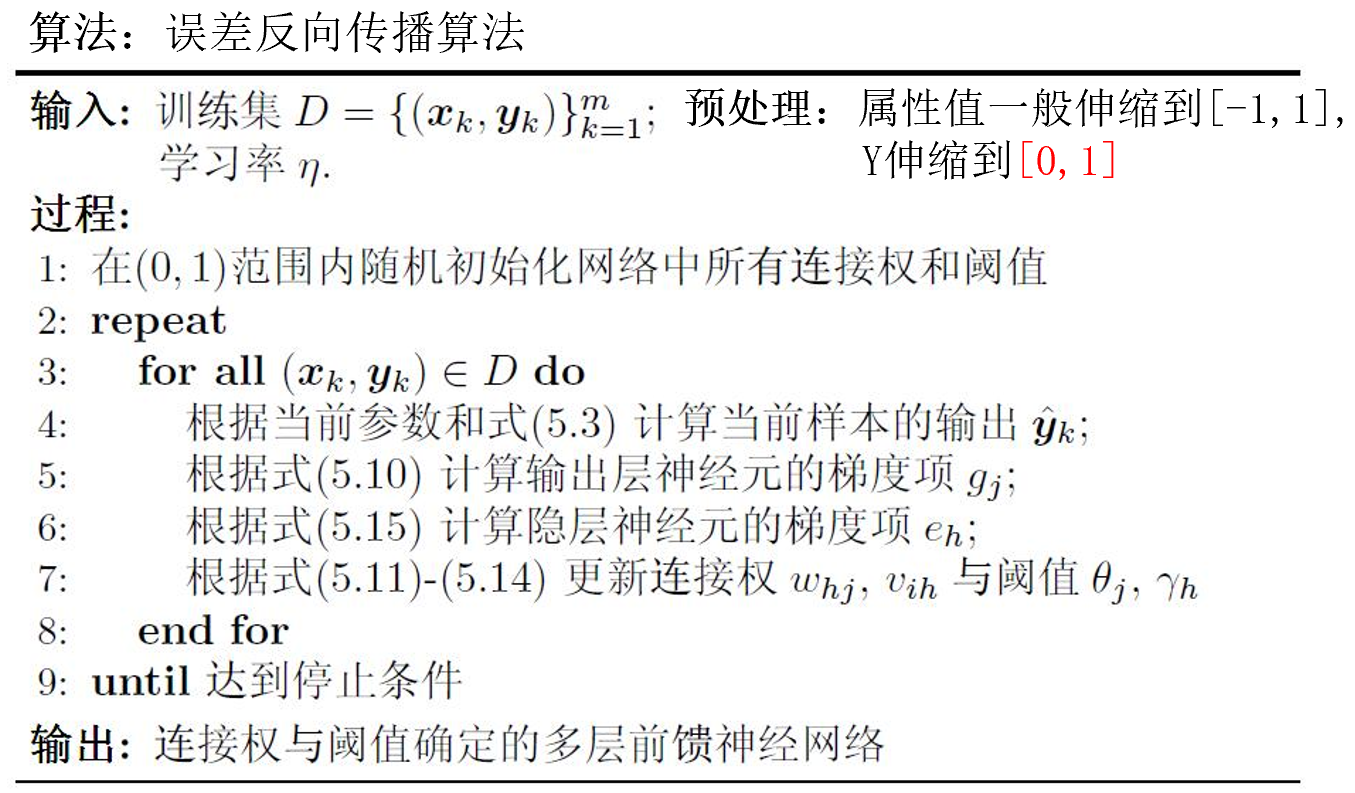## 累计误差反向传播算法

• 每次针对单个训练样例更新权值与阈值

• 参数更新频繁，不同样例可能抵消，需要多次迭代

• 其优化目标是最小化整个训练集上的累计误差

• 读取整个训练集一遍才对参数进行更新 , 参数更新

## 过拟合

BP网络经常出现过拟合现象。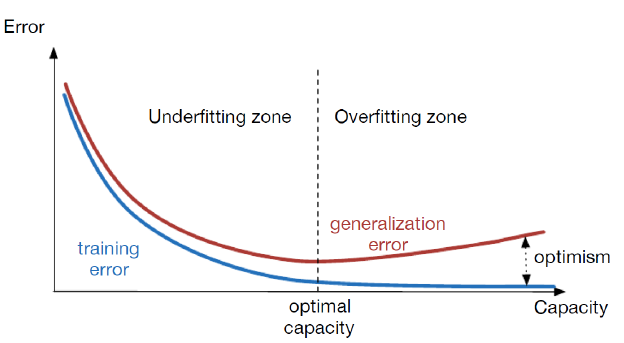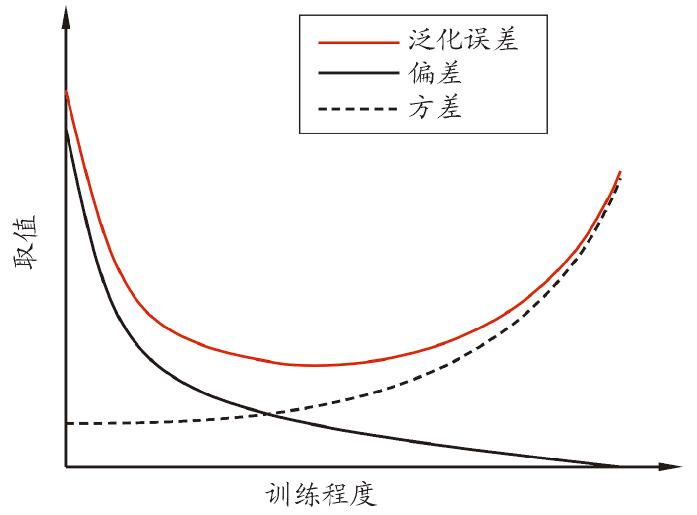1. 确保训练数据集和测试数据集是independent, identical distributed(i.i.d)的；

2. 早停（ early stopping ）：若训练集误差降低，但验证集误差升高，则停止训练；

3. 正则化（ regularization ：在误差目标函数中增加一个用于描述网络复杂度的部分。例如：

## 全局最小与局部最小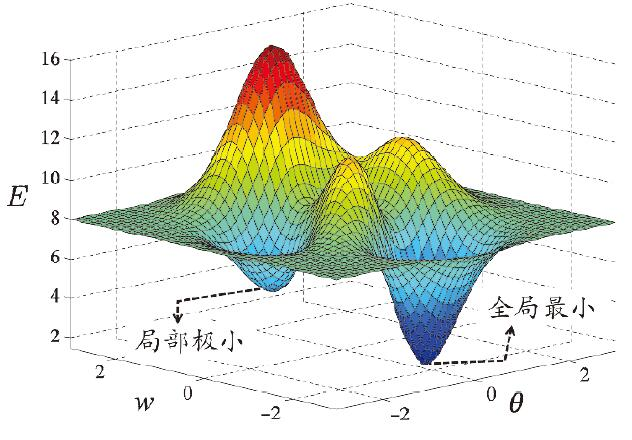“跳出”局部极小的常见策略：

• 不同的初始参数

• 模拟退火

• 随机扰动

• 遗传算法

• ……